In the formula . Ifthen find

# In the formula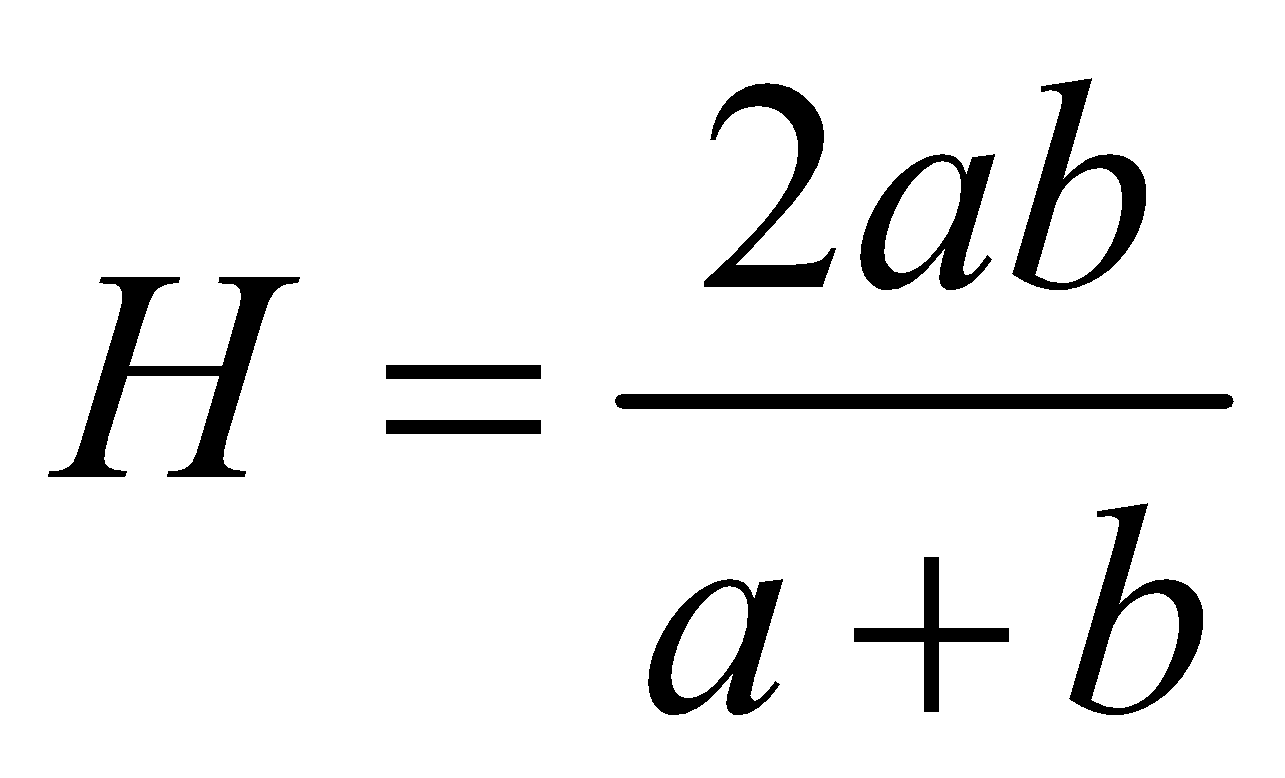. If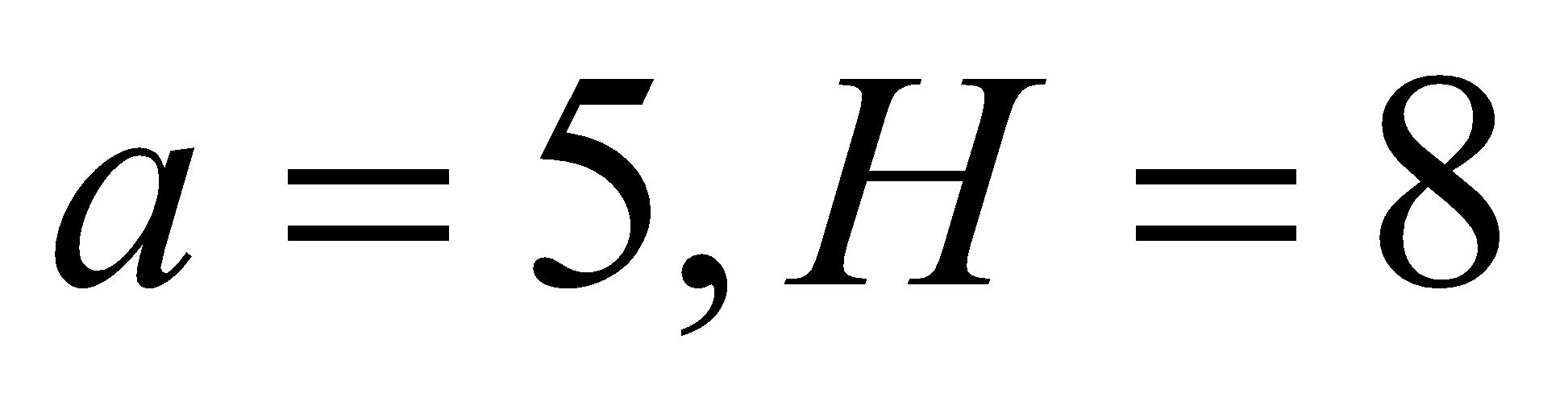then find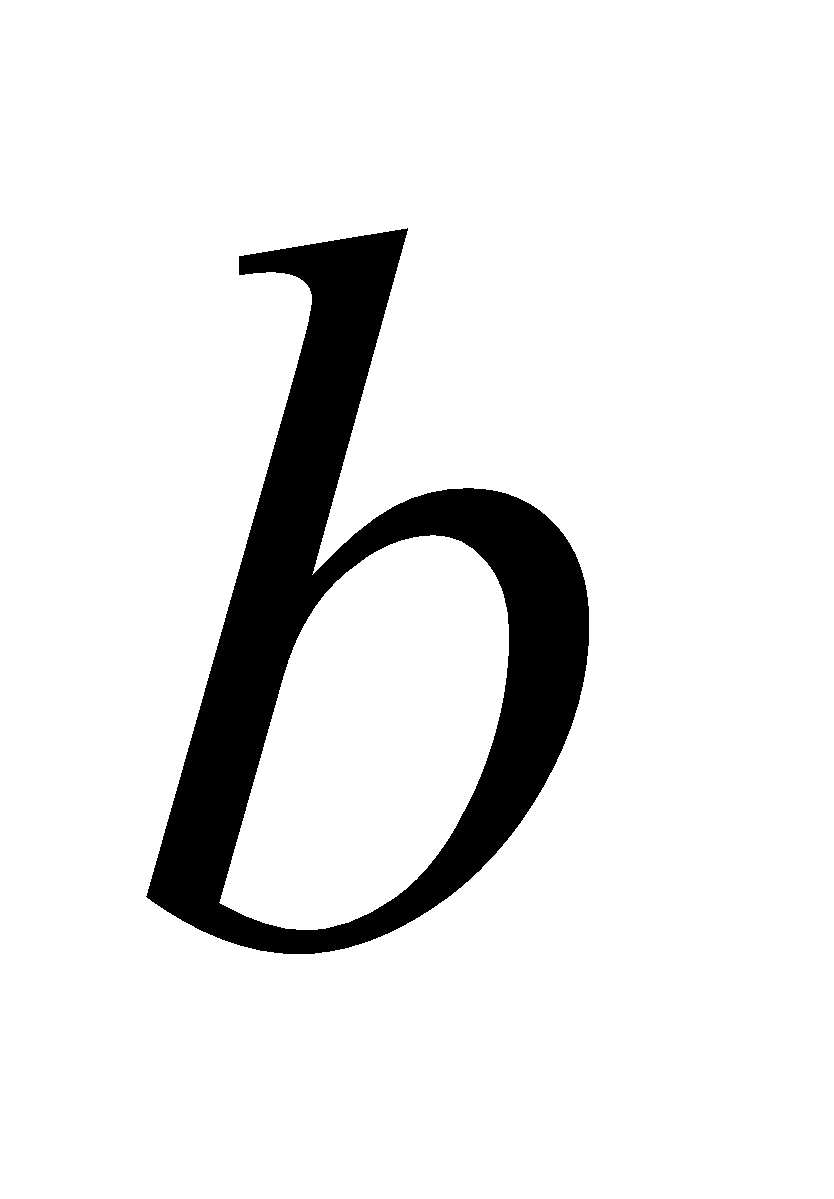1. A
10
2. B
20
3. C
30
4. D
25

Fill Out the Form for Expert Academic Guidance!l

+91

Live ClassesBooksTest SeriesSelf Learning

Verify OTP Code (required)

### Solution:

Concept- Here we solve them by putting the values ​​directly into the formula and adding, multiplying, subtracting, and dividing the numbers.
The formula given in the question isValues given areBy adjusting the value provided in the formula, we obtain-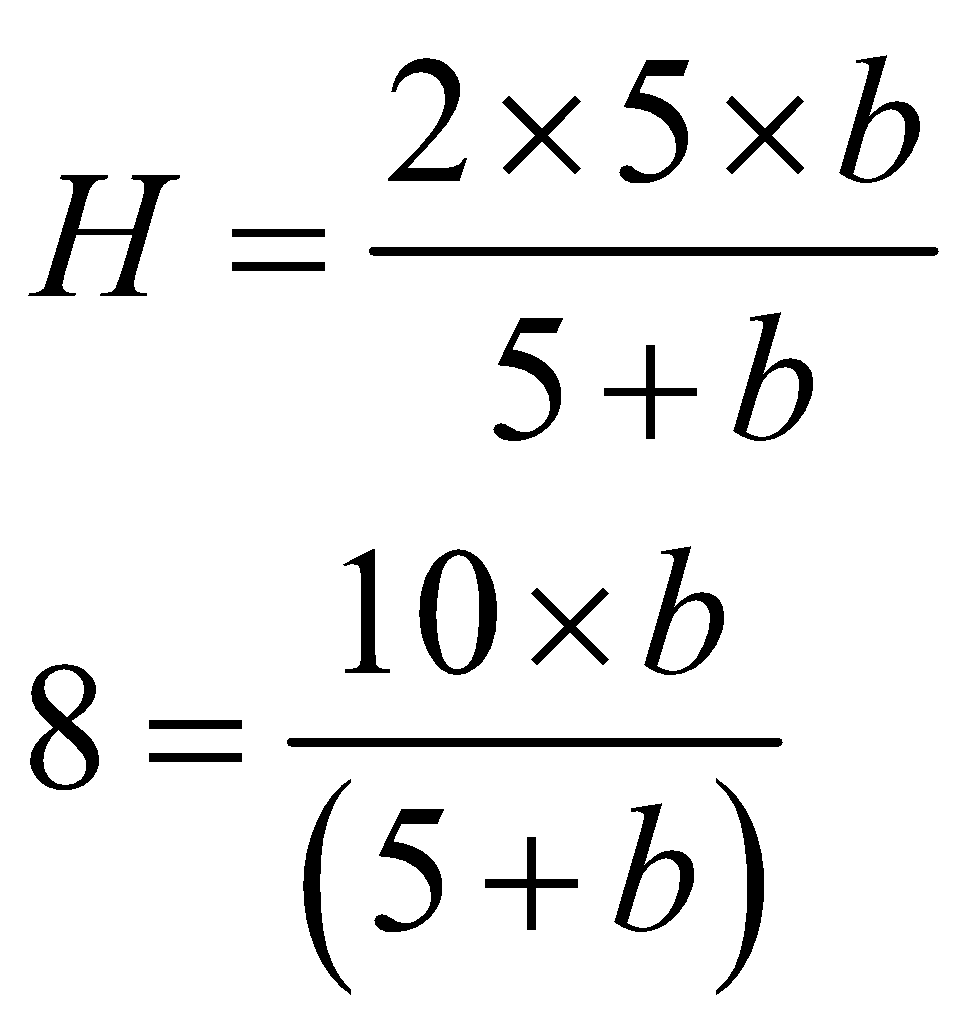By applying cross multiplication
We get,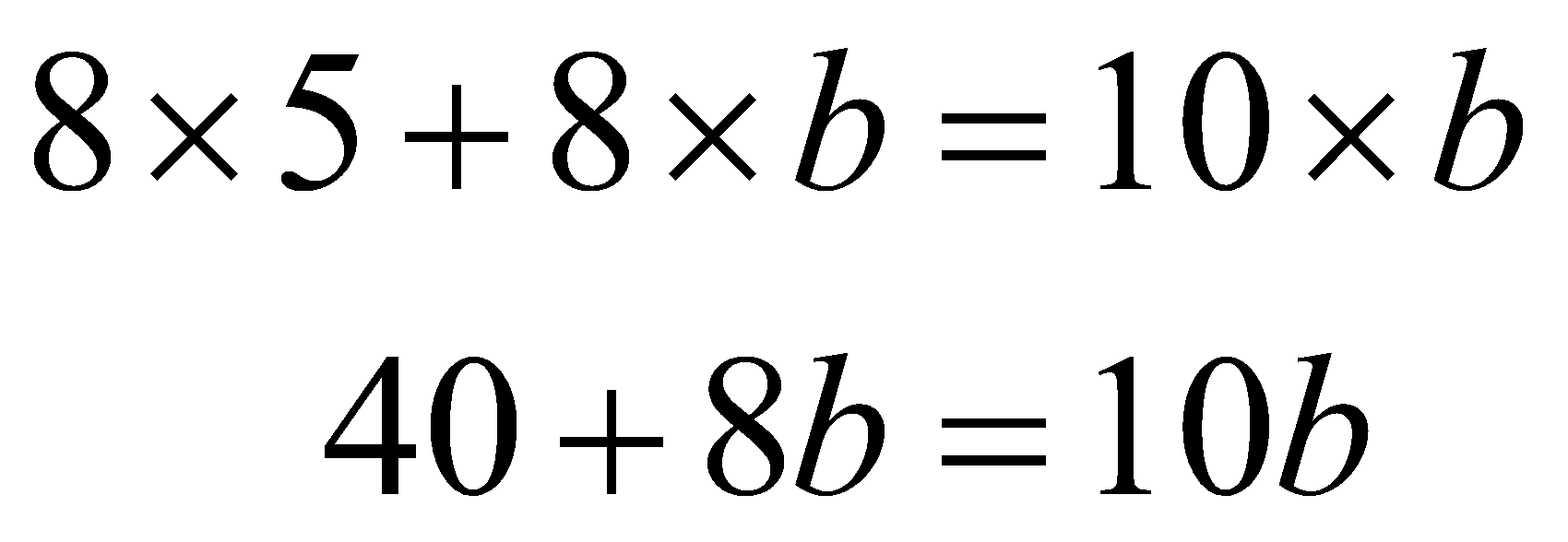Moving an expression containing a variable to one side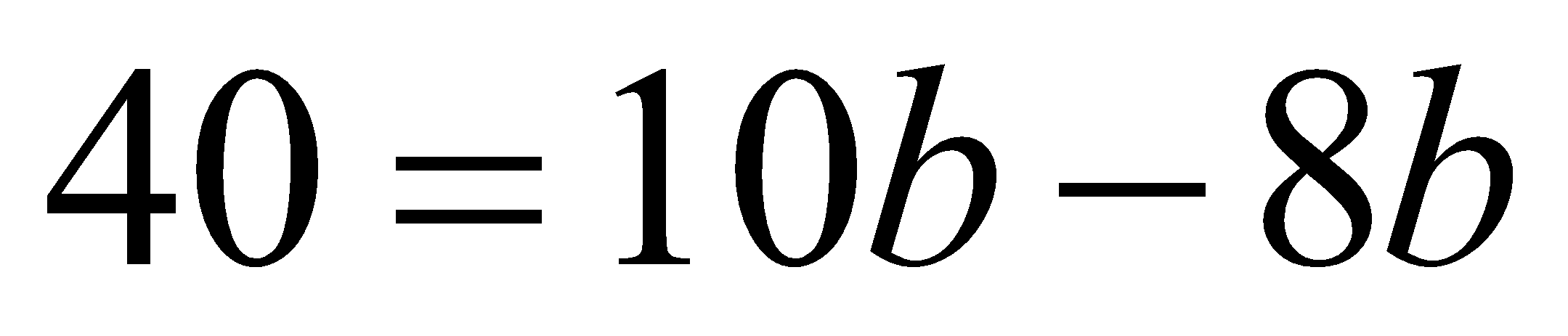By taking b common on right-hand side of the equation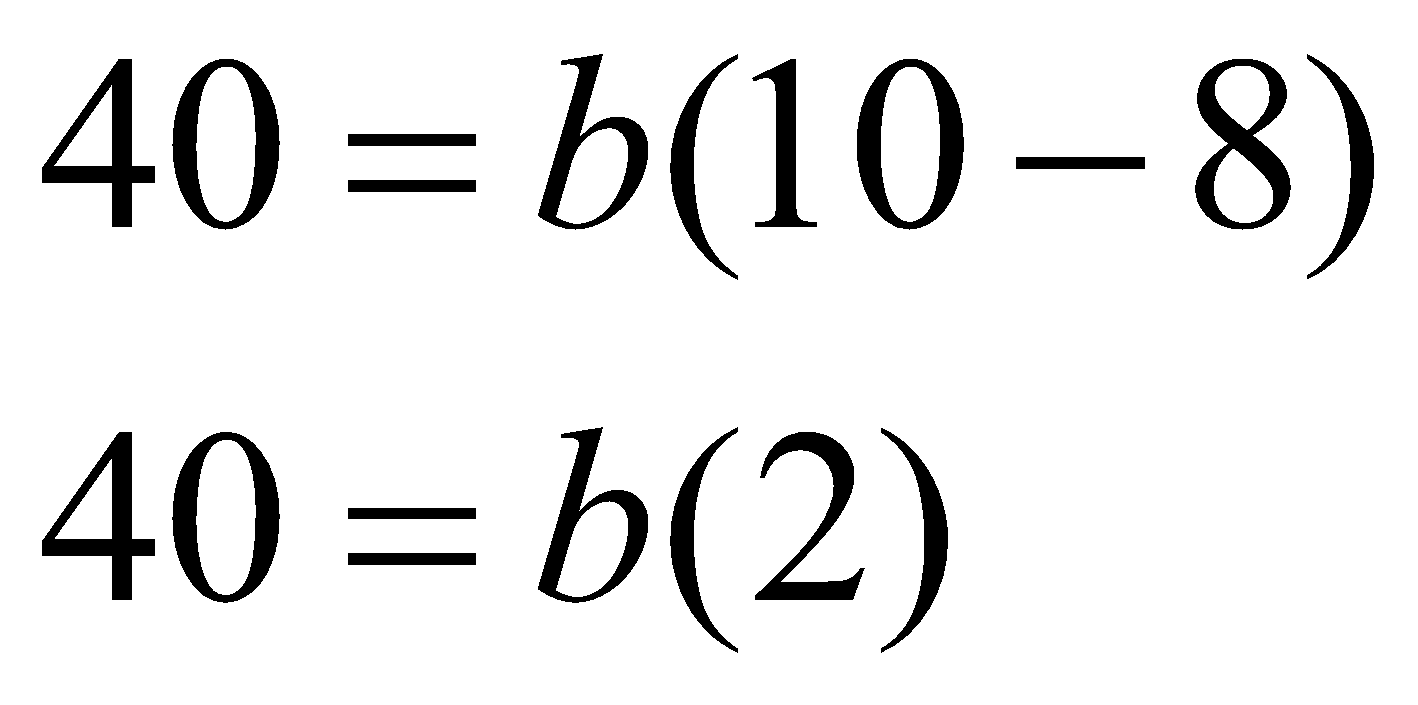Above Equation is divided by 2, then canceling out the same terms in denominator and numerator from both side of the equation gives: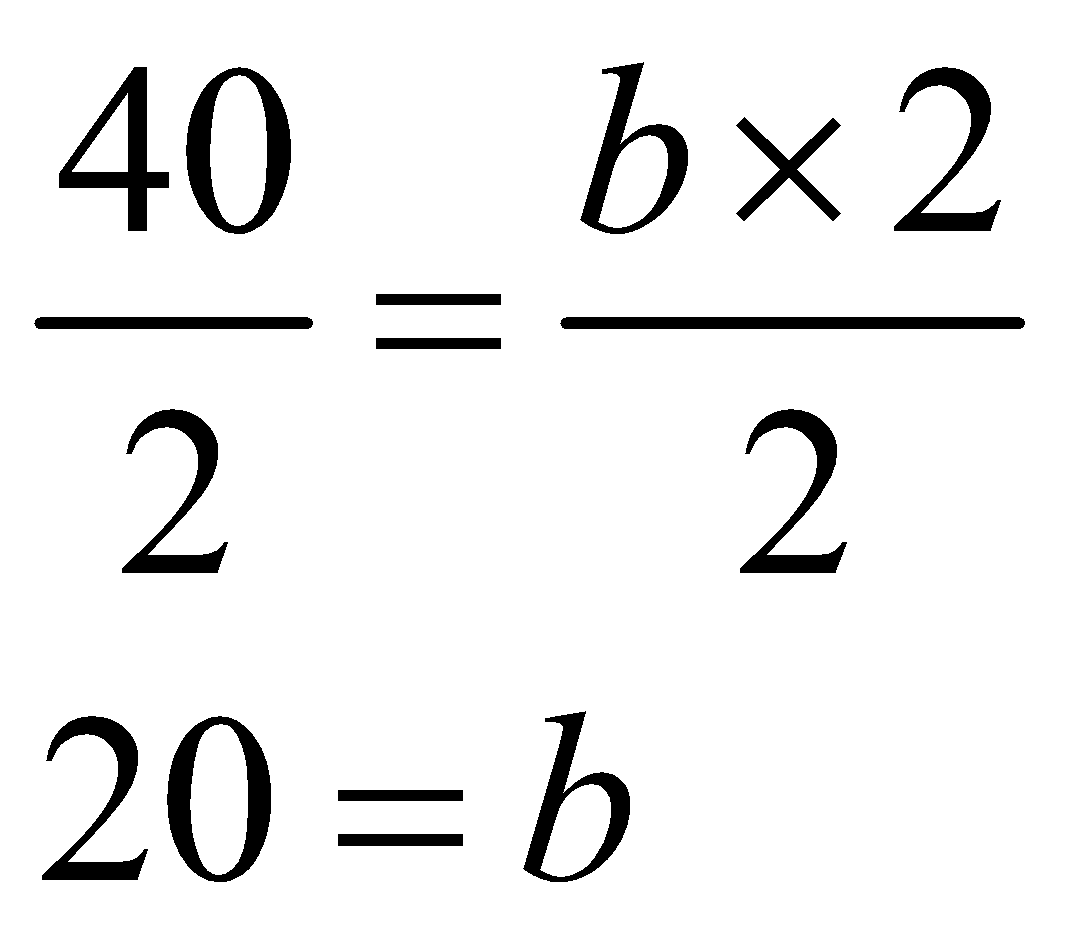Hence, the correct answer is option 2) 20

## Related content

 Area of Square Area of Isosceles Triangle Pythagoras Theorem Triangle Formula Perimeter of Triangle Formula Area Formulae Volume of Cone Formula Matrices and Determinants_mathematics Critical Points Solved Examples Type of relations_mathematics+91

Live ClassesBooksTest SeriesSelf Learning

Verify OTP Code (required)